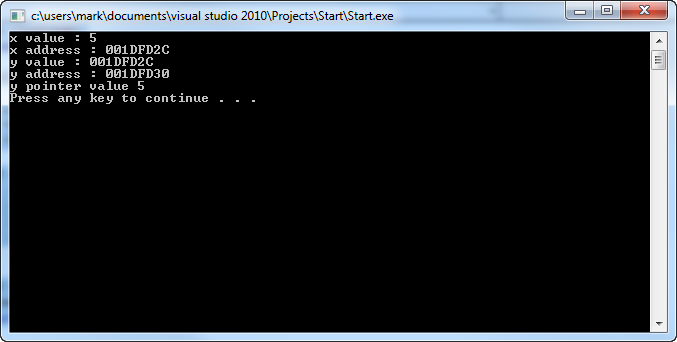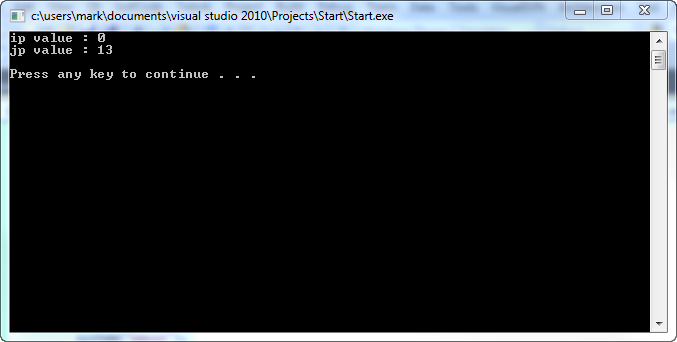Geeks With Blogs

Ever since I began programming in c++ I have gotten confused with how pointers work. I understand why they are there and why they can be useful, but in my managed world I really don’t like touching them…

That being said, tomorrow I am going to be tested on pointers and so I thought I would brush up.. here is a bit of sample code on some basic uses…

```#include <iostream>
using namespace std;

int main(int argc, char *argv[])
{
int x = 5;
int *y = &x;
int *z = y;

cout << "x value : " << x << endl
<< "x address : " << &x << endl
<< "y value : " << y << endl
<< "y address : " << &y << endl
<< "y pointer value " << *y;

cout << endl;
system("pause");
return 0;
}```

This gives the following output…One can also assign space on the heap for objects using the new keyword. Again, it is important that if you declare space using the new keyword you also call the delete method after you are finished so that space is freed up off the heap.

```    int main(int argc, char *argv[])
{
int *ip;
ip = new int();
int *jp = new int(13);

cout << "ip value : " << *ip << endl
<< "jp value : " << *jp << endl;

delete ip;
delete jp;

cout << endl;
system("pause");
return 0;
}```Posted on Friday, May 20, 2011 2:03 AM C++ , UNISA COS 2144 Programming Contemporary Concepts | Back to top

Related Posts on Geeks With Blogs Matching Categories

Comments on this post: Playing with Pointers